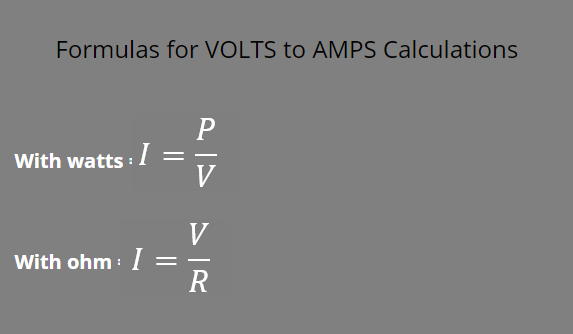(206) 687-4009 | [email protected]

# VOLTS to AMPS & AMPS to VOLTS CALCULATOR

In DC Circuits, it is easy to determine the voltage across a resistive load (by connecting a voltmeter in parallel with that resistance) but in a running circuit it is not easy to determine current using a normal ammeter and sometimes a resistive load power consumption and rated voltage is mentioned on device nameplate but we need to know its current (amps). So, volts to amps conversion calculator can help us in both cases. Similar types of possibilities can also appear for amps to volts conversion.

Choose what to calculate

Connection Type

Volts(V)
Volts
Power(P)
WattsChoose what to calculate

Connection Type

Current(I)
Amps
Power(P)
Watts## What are volts?

Volts are the SI unit of potential difference or voltage. it is defined as:

When 1 joule energy is required to move a charge of 1 coulomb it is equal to 1 volt.

## What are amps?

Amps are the SI unit of current. It is defined as:

When 1 coulomb charge moves for 1 sec it is equal to 1 amp.

## Ohm’s law:

Ohm’s law shows the relation between voltage and current. It is defined as:

‘The amount of current flowing through a conductor depends directly on potential difference across it.”

Final form of equation comes out to be:

V = I * R

Where:

• V=potential difference or voltage
• I=current
• R=resistance

## What are ohms?

Ohms are the SI unit of resistance. it is defined as:

When one ampere current is subjected to a potential difference of one volt.

## Volts to Amps or Amps to volts calculator parameter:

• Choose what to calculate: Volts to Amps or Amps to Volts
• Option: through power (watts) or resistance (ohms)
• If power selected: power to be provided in watts
• If resistance selected: resistance to be provided in ohms

## Converting Volts to Amps:

When resistance (ohms) is given:

The formula for volts to amps conversion when resistance is given:

I =
V / R

When power (watts) is given:

The formula for volts to amps conversion when power is given:

I =
P(watts) / V

## Converting Amps to Volts:

When resistance is given:

The formula for amps to volts conversion when resistance is given:

V = I * R

When power (watts) is given:

The formula for amps to volts conversion when power is given:

V =
P / I

## Solved Example:

### For volts to amps conversion:

When resistance is given:

Consider a DC system that has the following data:

Given:

Voltage =120 V

Resistance (R) = 30 ohms

Required:

Current (I) =? (Amps)

Solution:

From the formula of volts to amps conversion when resistance is given:

I =
120 / 30
=4 Amps

When power is given:

Consider a DC system that has the following data:

Given:

Voltage =120 V

Power (P) = 180 Watts

Required:

Current (I) =? (Amps)

Solution:

From the formula of volts to amps conversion when power is given:

I =
180 / 120
=1.5 Amps

### For amps to volts conversion:

When resistance is given:

Consider a DC system that has the following data:

Given:

Current (I) = 4 Amps

Resistance (R) = 30 ohms

Required:

Voltage (V) =? (Volts)

Solution:

From the formula of amps to volts conversion when resistance is given:

V =
4 * 30 /
=120 Volts

When power is given:

Consider a DC system that has the following data:

Given:

Current (I) =2 Amps

Power (P) = 180 Watts

Required:

Voltage (V) =? (Volts)

Solution:

From the formula of amps to volts conversion when power is given:

V =
180 / 2
=90 Volts